International
Tables for
Crystallography
Volume D
Physical properties of crystals
Edited by A. Authier

International Tables for Crystallography (2006). Vol. D, ch. 1.5, p. 146

Table 1.5.10.1

A. S. Borovik-Romanova and H. Grimmerb*

aP. L. Kapitza Institute for Physical Problems, Russian Academy of Sciences, Kosygin Street 2, 119334 Moscow, Russia, and bLabor für Neutronenstreuung, ETH Zurich, and Paul Scherrer Institute, CH-5234 Villigen PSI, Switzerland
Correspondence e-mail:  hans.grimmer@psi.ch

 Table 1.5.10.1| top | pdf | Conversion of Gaussian to SI units
SymbolQuantityGaussian unit and its SI equivalent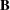Magnetic induction 1 gauss (G) = 10−4 tesla (T)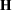Magnetic field 1 oersted (Oe) = 103/(4π) A m−1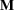Magnetization (= magnetic moment per unit volume) 1 emu cm−3 = 103 A m−1Linear magnetoelectric tensor (rationalized units) 1 (dimensionless units) = 4π × 10−8/3 s m−1Piezomagnetic tensor 1 Oe−1 = 4π × 10−3 m A−1 = 4π × 10−3 T Pa−1Magnetic volume susceptibility 1 (dimensionless units) = 4π (dimensionless units)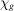Magnetic mass susceptibility 1 cm3 g−1 = 4π × 10−6 m3 g−1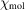Magnetic molar susceptibility 1 cm3 mol−1 = 4π × 10−6 m3 mol−1